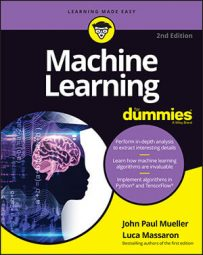##### Machine Learning For DummiesAlthough statistics are based on the expectation that features have certain value distributions, machine learning generally doesn’t have such constraints. A machine learning algorithm doesn’t need to know beforehand the type of data distribution it will work on, but learns it directly from the data used for training.

In probability, a distribution is a table of values or a mathematical function that links every possible value of a variable to the probability that such value could occur. Probability distributions are usually (but not solely) represented in charts whose abscissa axis represents the possible values of the variable and whose ordinal axis represents the probability of occurrence. Most statistical models rely on a normal distribution, a distribution that is symmetric and has a characteristic bell shape.

Even when using learning algorithms lent from statistics, you never need to transform an actual distribution to resemble a normal distribution or any other notable statistical distribution (such as uniform or Poisson distributions). Machine learning algorithms are usually smart enough to find out how to deal with any distribution present in the features by themselves. However, even if transforming the actual distributions isn’t necessary for a machine learning algorithm to work properly, it can still be beneficial for these reasons:
• To make the cost function minimize better the error of the predictions
• To make the algorithm converge properly and faster
You can apply transformations to the response variable to minimize the weight of any extreme case. When you predict values (as in a regression problem), and some of the values are too extreme with respect to the majority of values, you may apply transformations that tend to shorten the distance between values.

Consequently, when striving to minimize the error, the algorithm won’t focus too much on the extreme errors and will obtain a general solution. In such cases, you normally choose the logarithmic transformation, but this transformation requires positive values. If you work with just positive numbers and the problem is the zero, add 1 to your values so that none of them will be zero. (The result of log(1) is actually zero, making it a convenient new starting point.)

If you can’t use a logarithmic transformation, apply a cubic root, which preserves the sign. In certain cases (when the extreme values are huge), you may want to apply the inverse transformation instead (that is, divide 1 by the value of your response).

Some machine learning algorithms based on the gradient descent or on distance measures (like algorithms such as K-means and K-Nearest Neighbors) are quite sensitive to the scale of the numeric values provided. Consequently, in order for the algorithm to converge faster or to provide a more exact solution, rescaling the distribution is necessary. Rescaling mutates the range of the values of the features and can affect variance, too. You can perform features rescaling in two ways:
• Using statistical standardization (z-score normalization): Center the mean to zero (by subtracting the mean) and then divide the result by the standard deviation. After such transformation, you find most of the values in the range from –3 to +3.
• Using the min-max transformation (or normalization): Remove the minimum value of the feature and then divide by the range (maximum value minus minimum value). This act rescales all the values from 0 to 1. It’s preferred to standardization when the original standard deviation is too small (original values are too near, as if they all stick together around the mean) or when you want to preserve the zero values in a sparse matrix which explores the math of machine learning).
Both R and Python provide functions for distribution transformation. Apart from the mathematical functions log and exp, which are immediately available in R and require the import of the NumPy package in Python, standardization and min-max normalization require a little more work.

In R, you achieve standardization using the scale function (try `help(scale)` on R), but min-max normalization requires you to define your own function:

`min_max <- function(x)`

`{return ((x - min(x, na.rm=TRUE)) /`

`(max(x, na.rm=TRUE) - min(x, na.rm=TRUE)))}`

In Python, you use functions and classes from Scikit-learn module preprocessing, such as: `sklearn.preprocessing.scale, sklearn.preprocessing.StandardScaler`, and `sklearn.preprocessing.MinMaxScaler`.